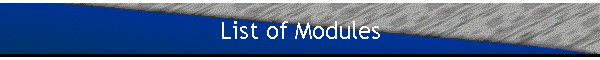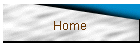• #### A Particle in a Central Field

• The asymptotic behavior of the radial functions, two interacting particles.
• #### Hydrogenic Systems

• The hydrogen atom, eigenvalues and eigenfunctions, spectroscopic notation, hydrogenic systems.
• #### Diatomic Molecules

• Motion of the nuclei, vibrational-rotational levels.
• #### Addition of Angular Momentum

• Clebsch-Gordan coefficients, adding more than two angular momenta, rotation matrices, transformation properties of the spherical harmonics, the Wigner-Eckart theorem, the projection theorem.
• #### Gauge Transformations, Flux Quantization, Propagator

• Gauge transformations in electromagnetism, the Aharonov-Bohm effect, magnetic monopoles, propagator and path integrals.
• #### Periodic Systems

• Periodic and continuous systems, Electrons in a solid.
• #### Scattering

• Scattering by a central potential, free spherical waves, partial waves, phase shifts,   the scattering cross section near a resonance, scattering length and effective range, frame transformations, integral scattering equation for stationary states, the Born approximation, the Yukawa potential, the Coulomb potential.
• #### Approximation Methods for bound states

• Stationary perturbation theory, the perturbation of degenerate states, the variational method,
• #### The fine structure and hyperfine structure of H

• The Dirac equation, the fine structure Hamiltonian, the hyperfine structure Hamiltonian.
• #### Time Dependent Perturbation Theory

• Atoms interacting with an electromagnetic wave, transition selection rules
• #### Identical Particles

• Permutation operators, Slater Determinants, atomic spectra, coupling schemes